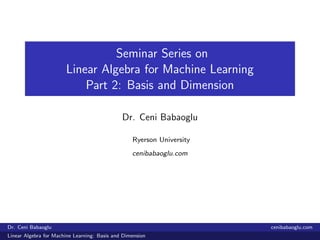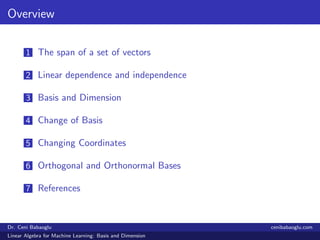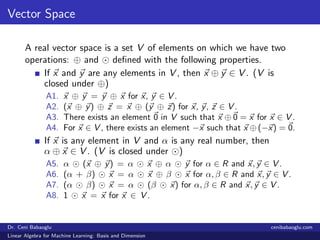Successfully reported this slideshow.

# 2. Linear Algebra for Machine Learning: Basis and Dimension

3

Share×
1 of 20
1 of 20

# 2. Linear Algebra for Machine Learning: Basis and Dimension

3

Share

The seminar series will focus on the mathematical background needed for machine learning. The first set of the seminars will be on "Linear Algebra for Machine Learning". Here are the slides of the second part which is discussing basis and dimension.
Here is the link of the first part which was discussing linear systems: https://www.slideshare.net/CeniBabaogluPhDinMat/linear-algebra-for-machine-learning-linear-systems/1

The seminar series will focus on the mathematical background needed for machine learning. The first set of the seminars will be on "Linear Algebra for Machine Learning". Here are the slides of the second part which is discussing basis and dimension.
Here is the link of the first part which was discussing linear systems: https://www.slideshare.net/CeniBabaogluPhDinMat/linear-algebra-for-machine-learning-linear-systems/1

## More Related Content

### Related Books

Free with a 14 day trial from Scribd

See all

### Related Audiobooks

Free with a 14 day trial from Scribd

See all

### 2. Linear Algebra for Machine Learning: Basis and Dimension

1. 1. Seminar Series on Linear Algebra for Machine Learning Part 2: Basis and Dimension Dr. Ceni Babaoglu Ryerson University cenibabaoglu.com Dr. Ceni Babaoglu cenibabaoglu.com Linear Algebra for Machine Learning: Basis and Dimension
2. 2. Overview 1 The span of a set of vectors 2 Linear dependence and independence 3 Basis and Dimension 4 Change of Basis 5 Changing Coordinates 6 Orthogonal and Orthonormal Bases 7 References Dr. Ceni Babaoglu cenibabaoglu.com Linear Algebra for Machine Learning: Basis and Dimension
3. 3. Vector Space A real vector space is a set V of elements on which we have two operations: ⊕ and deﬁned with the following properties. If x and y are any elements in V , then x ⊕ y ∈ V . (V is closed under ⊕) A1. x ⊕ y = y ⊕ x for x, y ∈ V . A2. (x ⊕ y) ⊕ z = x ⊕ (y ⊕ z) for x, y, z ∈ V . A3. There exists an element 0 in V such that x ⊕ 0 = x for x ∈ V . A4. For x ∈ V , there exists an element −x such that x ⊕ (−x) = 0. If x is any element in V and α is any real number, then α ⊕ x ∈ V . (V is closed under ) A5. α (x ⊕ y) = α x ⊕ α y for α ∈ R and x, y ∈ V . A6. (α + β) x = α x ⊕ β x for α, β ∈ R and x, y ∈ V . A7. (α β) x = α (β x) for α, β ∈ R and x, y ∈ V . A8. 1 x = x for x ∈ V . Dr. Ceni Babaoglu cenibabaoglu.com Linear Algebra for Machine Learning: Basis and Dimension
4. 4. The span of a set of vectors The set of all linear combinations of a set of vectors {v1, v2, · · · , vn} is called the span. Let S = {v1, v2, · · · , vn} be a subset of a vector space V . We say S spans V , if every vector in V can be written as a linear combination of vectors in S: v = c1v1 + c2v2 + · · · + cnvn. Note: A linear combination is in the form c1v1 + c2v2 + · · · + cnvn where ci ’s are real numbers. Dr. Ceni Babaoglu cenibabaoglu.com Linear Algebra for Machine Learning: Basis and Dimension
5. 5. Linear dependence and independence The vectors {v1, v2, · · · , vn}. are linearly dependent if there exist scalars, ci , i = 1, 2, · · · , n, not all zero, such that c1v1 + c2v2 + · · · + cnvn = 0. The vectors {v1, v2, · · · , vn} are linearly independent if and only if ci = 0, i = 1, 2, · · · , n, is the only solution to c1v1 + c2v2 + · · · + cnvn = 0. Dr. Ceni Babaoglu cenibabaoglu.com Linear Algebra for Machine Learning: Basis and Dimension
6. 6. Basis The vectors v1, v2, · · · , vn form a basis for a vector space V if and only if (i) v1, v2, · · · , vn are linearly independent. (ii) v1, v2, · · · , vn span V. If {v1, v2, · · · , vn} is a spanning set for a vector space V , then any collection of m vectors in V, where m > n, is linearly dependent. If {v1, v2, · · · , vn} and {u1, u2, · · · , um} are both bases for a vector space V , then n = m. Dr. Ceni Babaoglu cenibabaoglu.com Linear Algebra for Machine Learning: Basis and Dimension
7. 7. Dimension If a vector space V has a basis consisting of n vectors, we say that V has dimension n. If V is a vector space of dimension n, then (I) Any set of n linearly independent vectors spans V . (II) Any n vectors that span V are linearly independent. (III) No set of less than n vectors can span V . (IV) Any subset of less than n linearly independent vectors can be extended to form a basis for V . (V) Any spanning set containing more than n vectors can be pared down to form a basis for V . Dr. Ceni Babaoglu cenibabaoglu.com Linear Algebra for Machine Learning: Basis and Dimension
8. 8. Example Rn is n-dimensional. Let x = [x1 x2 x3 · · · xn]T ∈ Rn. Then      x1 x2 ... xn      = x1        1 0 0 ... 0        + x2        0 1 0 ... 0        + · · · + xn        0 0 0 ... 1        ≡ x1i1 + x2i2 + · · · + xnin. Therefore span{i1, i2, · · · , in} = Rn. i1, i2, · · · , in are linearly independent. They form a basis and this implies the dimension of R is n. Dr. Ceni Babaoglu cenibabaoglu.com Linear Algebra for Machine Learning: Basis and Dimension
9. 9. Note The basis in the previous example is the standard basis for Rn. In R3 the following notation is used for the standard basis: i =   1 0 0   , j =   0 1 0   , k =   0 0 1   . In an n-dimensional vector space V , set of n elements that span V must be independent and any set of n independent elements must span V . Dr. Ceni Babaoglu cenibabaoglu.com Linear Algebra for Machine Learning: Basis and Dimension
10. 10. Change of Basis The standard basis for R2 is {e1, e2}. Any vector x ∈ R2 can be expressed as a linear combination x = x1e1 + x2e2. The scalars x1 and x2 can be thought of as the coordinates of x with respect to the standard basis. For any basis {y, z} for R2, a given vector x can be represented uniquely as a linear combination, x = αy + βz. [y, z]: ordered basis (α, β)T : the coordinate vector of x with respect to [y, z] If we reverse the order of the basis vectors and take [z, y], then we must also reorder the coordinate vector and take (β, α)T . Dr. Ceni Babaoglu cenibabaoglu.com Linear Algebra for Machine Learning: Basis and Dimension
11. 11. Example y = (2, 1)T and z = (1, 4)T are linearly independent and form a basis for R2. x = (7, 7)T can be written as a linear combination: x = 3y + z The coordinate vector of x with respect to [y, z] is (3, 1)T . Geometrically, the coordinate vector speciﬁes how to get from the origin O(0, 0) to the point P(7, 7), moving ﬁrst in the direction of y and then in the direction of z . Dr. Ceni Babaoglu cenibabaoglu.com Linear Algebra for Machine Learning: Basis and Dimension
12. 12. Changing Coordinates Suppose, for example, instead of using [e1, e2] for R2, we wish to use a diﬀerent basis, say u1 = 3 2 , u2 = 1 1 . I. Given a vector c1u1 + c2u2, let’s ﬁnd its coordinates with respect to e1 and e2. II. Given a vector x = (x1, x2)T , let’s ﬁnd its coordinates with respect to u1 and u2. Dr. Ceni Babaoglu cenibabaoglu.com Linear Algebra for Machine Learning: Basis and Dimension
13. 13. Changing Coordinates I. Given a vector c1u1 + c2u2, let’s ﬁnd its coordinates with respect to e1 and e2. u1 = 3e1 + 2e2, u2 = e1 + e2 c1u1 + c2u2 = (3c1e1 + 2c1e2) + (c2e1 + c2e2) = (3c1 + c2)e1 + (2c1 + c2)e2 x = 3c1 + c2 2c1 + c2 = 3 1 2 1 c1 c2 U = (u1, u2) = 3 1 2 1 U: the transition matrix from [u1, u2] to [e1, e2] Given any coordinate c with respect to [u1, u2], the corresponding coordinate vector x with respect to [e1, e2] by x = Uc Dr. Ceni Babaoglu cenibabaoglu.com Linear Algebra for Machine Learning: Basis and Dimension
14. 14. Changing Coordinates II. Given a vector x = (x1, x2)T , let’s ﬁnd its coordinates with respect to u1 and u2. We have to ﬁnd the transition matrix from [e1, e2] to [u1, u2]. The matrix U is nonsingular, since its column vectors u1 and u2 are linearly independent. c = U−1 x Given vector x, x = (x1, x2)T = x1e1 + x2e2 We need to multiply by U−1 to ﬁnd its coordinate vector with respect to [u1, u2]. U−1: the transition matrix from [e1, e2] to [u1, u2] Dr. Ceni Babaoglu cenibabaoglu.com Linear Algebra for Machine Learning: Basis and Dimension
15. 15. Example Let E = (1, 2)T , (0, 1)T and F = (1, 3)T , (−1, 2)T be ordered basis for R2. (i) Find the transition matrix S from the basis E to the basis F. (ii) If the vector x ∈ R2 has the coordinate vector [x]E = (5, 0)T with respect to the ordered basis E, determine the coordinate vector [x]F with respect to the ordered basis F. Dr. Ceni Babaoglu cenibabaoglu.com Linear Algebra for Machine Learning: Basis and Dimension
16. 16. Example (i) We should ﬁnd the matrix S = a c b d such that [x]F = S [x]E for x ∈ R2. We solve the following system: 1 2 = a 1 3 + b −1 2 0 1 = c 1 3 + d −1 2 ⇒ 1 = a − b 2 = 3a + 2b 0 = c − d 1 = 3c + 2d ⇒ a = 4/5 b = −1/5 c = d = 1/5 ⇒ S = 4/5 1/5 −1/5 1/5 Dr. Ceni Babaoglu cenibabaoglu.com Linear Algebra for Machine Learning: Basis and Dimension
17. 17. Example E -V (1, 0)T , (0, 1)T ? U−1 Z Z Z Z Z Z Z ZZ~ U−1V F where V = 1 0 2 1 and U = 1 −1 3 2 . Thus we obtain U−1 = 2/5 1/5 −3/5 1/5 and then S = U−1 V = 4/5 1/5 −1/5 1/5 . Dr. Ceni Babaoglu cenibabaoglu.com Linear Algebra for Machine Learning: Basis and Dimension
18. 18. Example (ii) Since [x]E = (5, 0)T , [x]F = S [x]E = 4/5 1/5 −1/5 1/5 5 0 = 4 −1 Dr. Ceni Babaoglu cenibabaoglu.com Linear Algebra for Machine Learning: Basis and Dimension
19. 19. Orthogonal and Orthonormal Bases n linearly independent real vectors span Rn and they form a basis for the space. An orthogonal basis, a1, · · · , an satisﬁes ai · aj = 0, if i = j An orthonormal basis, a1, · · · , an satisﬁes ai · aj = 0, if i = j ai · aj = 1, if i = j Dr. Ceni Babaoglu cenibabaoglu.com Linear Algebra for Machine Learning: Basis and Dimension
20. 20. References Linear Algebra With Applications, 7th Edition by Steven J. Leon. Elementary Linear Algebra with Applications, 9th Edition by Bernard Kolman and David Hill. Dr. Ceni Babaoglu cenibabaoglu.com Linear Algebra for Machine Learning: Basis and Dimension